# Weight

## Key Stage 2

### Meaning

Weight is how heavy or light something is.

Objects have weight because of the gravity of the Earth.
Weight can be measured using a Newton Meter or a Measuring Scale.
Weight is measured in Newtons but can also be measured in stone, pounds and ounces.
Weight depends on the amount of mass an object has.
 Golf Ball Beach Ball Bowling Ball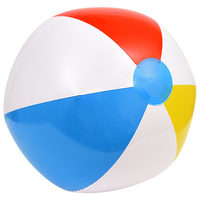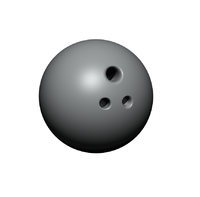The Golf Ball is not made of much material so it is lightest. The Beach Ball might be the biggest but it is not the heaviest. The Bowling Ball is made of the most material, so it is the heaviest.

## Key Stage 3

### Meaning

Weight is the the force on an object that is in a gravitational field.

Weight is a force so it is measured in Newtons.
Weight is a non-contact force because an object does not need to be touching the ground to be attracted to the Earth.
Weight always acts downwards.
All objects on Earth have weight because the Earth has a gravitational field.
Different planets have a different strength gravitational field, so the same object might weigh a different amount on different planets.
On Earth the gravitational field strength is roughly 10N/kg

### Examples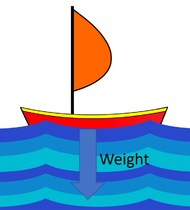Weight makes a tennis ball fall to the ground. Weight holds the bowling ball to the ground. If the weight of a boat were bigger than the upthrust the boat would sink.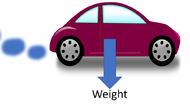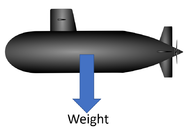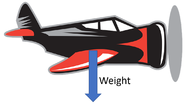The weight of the car acts from its centre of mass. The submarine has the same weight under the water as it would on land, but in the water this is balanced by the upthrust. Planes can be very heavy but their weight is balanced by the lift.

### Equation

Weight = Mass x Gravitational Field Strength

$$W = m \times g$$

Where:

W = Weight
m = Mass
g = Gravitational Field Strength

### Example Calculations

 Calculate the weight of a 25kg object on Earth. A 550kg space probe is sent into deep space beyond the gravitational field of any other object. Calculate the weight of the space probe. The Moon has a gravitational field strength of 1.6N/kg. Calculate the weight of a 85kg astronaut on The Moon. m = 25kg gEarth = 10N/kg $$W = m \times g$$ $$W = 25 \times 10$$ $$W = 250N$$ m = 550kg gDeep Space = 0N/kg $$W = m \times g$$ $$W = 550 \times 0$$ $$W = 0N$$ m = 85kg gThe Moon = 1.6N/kg $$W = m \times g$$ $$W = 85 \times 1.6$$ $$W = 136N$$

## Key Stage 4

### Meaning

Weight is the force acting on an object in a gravitational field.

The weight of an object is due to gravity.

The magnitude of the weight depends on:

Weight always acts towards the centre of mass of a moon, planet or star.
The weight of an object acts from its centre of mass (or centre of gravity) of an object.
On Earth the gravitational field strength is 9.8N/kg correct to two significant figures.

### ExamplesWeight makes a tennis ball fall to the ground. Weight holds the bowling ball to the ground. If the weight of a boat were bigger than the upthrust the boat would sink.The weight of the car acts from its centre of mass. The submarine has the same weight under the water as it would on land, but in the water this is balanced by the upthrust. Planes can be very heavy but their weight is balanced by the lift.

### Equation

Weight = Mass x Gravitational Field Strength

$$W = m \times g$$

Where

$$W$$ = The weight of the object.

$$W = m \times g$$ = The mass of the object.

$$W = m \times g$$ = The gravitational field strength of the gravitational field the object is in.

### Calculating Weight from Mass and Gravitational Field Strength

 Calculate the weight of a 25kg object on Earth correct to two significant figures A 550kg space probe is sent into deep space beyond the gravitational field of any other object. Calculate the weight of the space probe correct to two significant figures. The Moon has a gravitational field strength of 1.6N/kg. Calculate the weight of a 85kg astronaut on The Moon correct to two significant figures. 1. State the known quantities in SI Units.m = 25kg gEarth = 9.8N/kg 1. State the known quantities in SI Units.m = 550kg gDeep Space = 0N/kg 1. State the known quantities in SI Units.m = 550kg m = 85kg gThe Moon = 1.6N/kg 2. Substitute the numbers into the equation and solve. $$W = m \times g$$ $$W = 25 \times 9.8$$ $$W = 245N$$ $$W \approx 250N$$ 2. Substitute the numbers into the equation and solve. $$W = m \times g$$ $$W = 550 \times 0$$ $$W = 0.0N$$ 2. Substitute the numbers into the equation and solve. $$W = m \times g$$ $$W = 85 \times 1.6$$ $$W = 136N$$ $$W \approx 140N$$